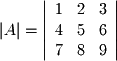A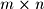matrix determinant requires a few more steps. It is computed by continuously breaking matrices down into smaller matrices until the 2x2 form is reached in a process called Expansion by Minors also known as Cofactor Expansion. We first define the minor matrix of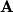as the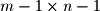matrix which is derived fromby eliminating the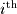row and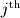column. For example, the 3x3 matrixand its minor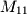(given by eliminating row 1 and column 1) are shown below: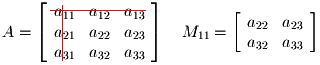The determinant ofis given by the following expansion by the first row of: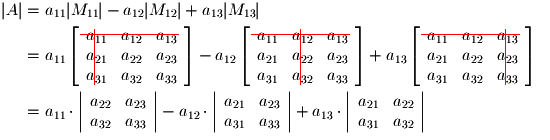The second term is negative in this expansion because the sign of the cofactors is given by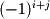. Taking these definitions together we can write the determinant by expansion along rowformally as follows: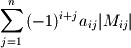It is valid to expand about any row or column. This can be especially useful if expansion about a particular row or column results in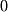value for one or more of the cofactors since this eliminates an entire minor matrix from the calculation.
Excercise 3-7.
Find the following matrix determinant: